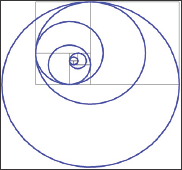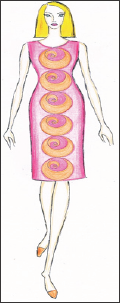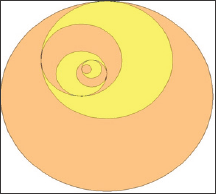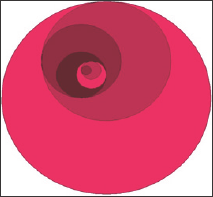## Editorial

Fibonacci circle is a pattern which is created on the base of Fibonacci spiral square tilling and Fibonacci spiral. Fibonacci circle is designed similarly to Fibonacci spiral using the frame of Fibonacci spiral square tilling. The difference between both designs is: The spiral is drawn with quarter-circle arcs inscribed in the squares and Fibonacci circle is constructed with whole circles . The design of Fibonacci circle on the frame of Fibonacci spiral square tilling is presented in Figure 1. The pattern without frame is shown in Figure 2. Similarly to Fibonacci circle the Golden circle can be created on the base of the Golden spiral .
Figure 1: Fibonacci circle, designed on the base of Fibonacci spiral square tilling.Figure 2: Fibonacci circle without the frame of Fibonacci spiral square tilling.Fibonacci circle can be applied in Fashion design similarly to other Fibonacci geometry creations as symbols of beauty and harmony.

## An Example for Application of Fibonacci Circle in Fashion Design

Figure 3 presents a design of a lady's dress with an application of Fibonacci circle. The circles are designed in one size and are arranged in a linear rhythm in vertical direction along the whole length of the dress. The front neckline is formed with a circle arc with diameter equal to the biggest of the circles in the used pattern of Fibonacci circle. Fibonacci circles are designed in two colors as both colors are alternated. Another bi-colors combination for Fibonacci circle is shown in Figure 4. The circles can be designed in four colors like it is presented in Figure 5 where the circles in every of the four directions are colored in particular hue.
Figure 3: A lady's dress with Fibonacci circles.Figure 4: Fibonacci circle in two colors.Figure 5: Fibonacci circle in four colors.## Conclusion

The Fibonacci circle can be applied in fashion design in variety of rhythms (linear, on the base of square and triangle sets, spiral one, and etc. according to the types of symmetry and proportions), various color combinations, and in different proportion and directions according to the clothing pieces. The other elements and pieces of the garments can be formed according to Fibonacci circle.